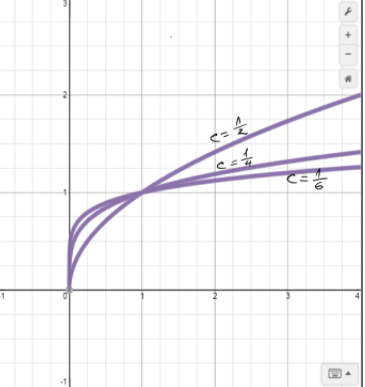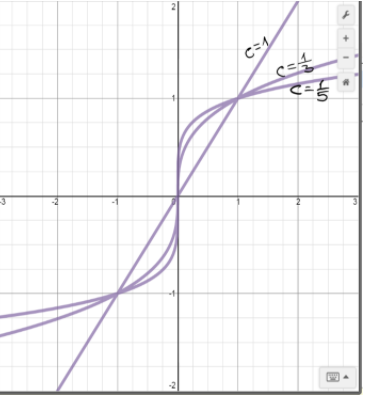# To graph: For the given member of family in viewing rectangle indicated.### Precalculus: Mathematics for Calcu...

6th Edition
Stewart + 5 others
Publisher: Cengage Learning
ISBN: 9780840068071### Precalculus: Mathematics for Calcu...

6th Edition
Stewart + 5 others
Publisher: Cengage Learning
ISBN: 9780840068071

#### Solutions

Chapter 2.2, Problem 73E

(a)

To determine

## To graph:For the given member of family in viewing rectangle indicated.

Expert Solution

### Explanation of Solution

Given information:

The given function is f(x)=xc .

The value of c is c=12,14,16;[1,4]by[1,3] .

Graph:

The graph for the given family of equations is shown in figure (1).Figure (1)

Interpretation: Graph for the family of equations of the function f(x)=xc is shown in figure (1).

(b)

To determine

Expert Solution

### Explanation of Solution

Given information:

The given function is f(x)=xc .

The value of c is c=1,13,15;[3,3]by[2,2] .

Graph:

The graph for the given family of equations is shown in figure (2).Figure (2)

Interpretation: Graph for the family of equations of the function f(x)=xc is shown in figure (2).

(c)

To determine

### To find: The conclusions from the graphs and effects on the value of c .

Expert Solution

The graphs of even roots are similar to y=x and for odd roots are similar to y=x3 .

### Explanation of Solution

Given information:

The given function is f(x)=xc .

Calculation:

From the graphs in part (a) and (b), we can observed that the graphs of even roots are similar to y=x .

The graphs of odd roots are similar to y=x3 .

As c increases

The graph of y=x1/c=xc becomes steeper near x=0 and flatter when x>1 .

Therefore, the graphs of even roots are similar to y=x and for odd roots are similar to y=x3 .

### Have a homework question?

Subscribe to bartleby learn! Ask subject matter experts 30 homework questions each month. Plus, you’ll have access to millions of step-by-step textbook answers!